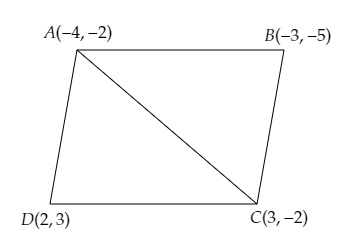# Find the area of the quadrilaterals, the coordinates of whose vertices are$(-4, -2), (-3, -5), (3, -2), (2, 3)$

Given:

Vertices of a quadrilateral are $(-4, -2), (-3, -5), (3, -2), (2, 3)$.

To do:

We have to find the area of the quadrilateral.

Solution:

Let $A (-4, -2), B (-3, -5), C(3,-2)$ and $D (2, 3)$ be the vertices of a quadrilateral $ABCD$.

Join $A$ and $C$ to get two triangles $ABC$ and $ADC$.This implies,

Area of quadrilateral $ABCD=$ Area of triangle $ABC+$ Area of triangle $ADC$.

We know that,

Area of a triangle with vertices $(x_1,y_1), (x_2,y_2), (x_3,y_3)$ is given by,

Area of $\Delta=\frac{1}{2}[x_{1}(y_{2}-y_{3})+x_{2}(y_{3}-y_{1})+x_{3}(y_{1}-y_{2})]$

Therefore,

Area of triangle $ABC=\frac{1}{2}[-4(-5+2)+(-3)(-2+2)+3(-2+5)]$

$=\frac{1}{2}[-4(-3)+(-3)0+3(3)]$

$=\frac{1}{2}[12+9]$

$=\frac{1}{2} \times 21$

$=\frac{21}{2}$ sq. units.

Area of triangle $ADC=\frac{1}{2}[-4(-2-3)+2(-2+2)+3(3+2)]$

$=\frac{1}{2}[-4(-5)+2(0)+3(5)]$

$=\frac{1}{2}[20+0+15]$

$=\frac{1}{2} \times 35$

$=\frac{35}{2}$ sq. units.

Therefore,

The area of the quadrilateral $ABCD=\frac{21}{2}+\frac{35}{2}=\frac{21+35}{2}=\frac{56}{2}=28$ sq. units.

The area of the given quadrilateral is $28$ sq. units.

Updated on: 10-Oct-2022

24 Views